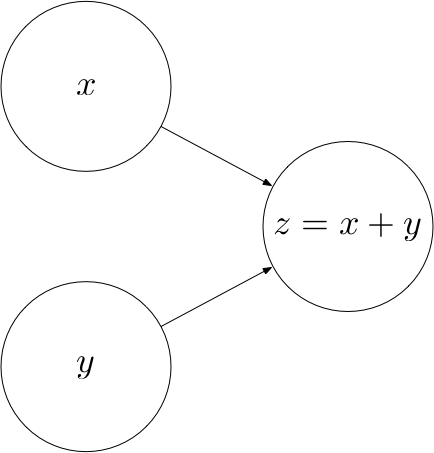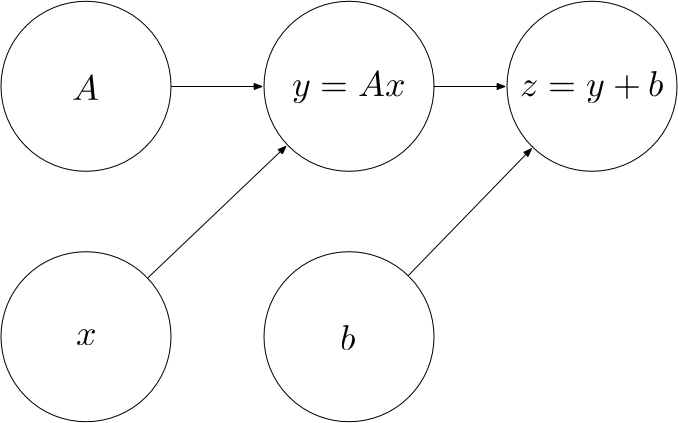This is part 1 of a series of tutorials, in which we develop the mathematical and algorithmic underpinnings of deep neural networks from scratch and implement our own neural network library in Python, mimicing the TensorFlow API.

I do not assume that you have any preknowledge about machine learning or neural networks. However, you should have some preknowledge of calculus, linear algebra, fundamental algorithms and probability theory on an undergraduate level. If you get stuck at some point, please leave a comment.

By the end of this text, you will have a deep understanding of the math behind neural networks and how deep learning libraries work under the hood.

I have tried to keep the code as simple and concise as possible, favoring conceptual clarity over efficiency. Since our API mimics the TensorFlow API, you will know how to use TensorFlow once you have finished this text, and you will know how TensorFlow works under the hood conceptually (without all the overhead that comes with an omnipotent, maximally efficient machine learning API).

The full source code of the API can be found at https://github.com/danielsabinasz/TensorSlow. You also find a Jupyter Notebook there, which is equivalent to this blog post but allows you to fiddle with the code.

# Computational graphs

We shall start by defining the concept of a computational graph, since neural networks are a special form thereof. A computational graph is a directed graph where the nodes correspond to operations or variables. Variables can feed their value into operations, and operations can feed their output into other operations. This way, every node in the graph defines a function of the variables.

The values that are fed into the nodes and come out of the nodes are called tensors, which is just a fancy word for a multi-dimensional array. Hence, it subsumes scalars, vectors and matrices as well as tensors of a higher rank.

Let’s look at an example. The following computational graph computes the sum $z$ of two inputs $x$ and $y$.
Here, $x$ and $y$ are input nodes to $z$ and $z$ is a consumer of $x$ and $y$. $z$ therefore defines a function $z : \mathbb{R^2} \rightarrow \mathbb{R}$ where $z(x, y) = x + y$.The concept of a computational graph becomes more useful once the computations become more complex. For example, the following computational graph defines an affine transformation $z(A, x, b) = Ax + b$.## Operations

Every operation is characterized by three things:

• A compute function that computes the operation’s output given values for the operation’s inputs
• A list of input_nodes which can be variables or other operations
• A list of consumers that use the operation’s output as their input

Let’s put this into code:

### Some elementary operations

Let’s implement some elementary operations in order to become familiar with the Operation class (and because we will need them later). In both of these operations, we assume that the tensors are NumPy arrays, in which the element-wise addition and matrix multiplication (.dot) are already implemented for us.

## Placeholders

Not all the nodes in a computational graph are operations. For example, in the affine transformation graph, $A$, $x$ and $b$ are not operations. Rather, they are inputs to the graph that have to be supplied with a value once we want to compute the output of the graph. To provide such values, we introduce placeholders.

## Variables

In the affine transformation graph, there is a qualitative difference between $x$ on the one hand and $A$ and $b$ on the other hand. While $x$ is an input to the operation, $A$ and $b$ are parameters of the operation, i.e. they are intrinsic to the graph. We will refer to such parameters as Variables.

## The Graph class

Finally, we’ll need a class that bundles all the operations, placeholders and variables together. When creating a new graph, we can call its as_default method to set the _default_graph to this graph. This way, we can create operations, placeholders and variables without having to pass in a reference to the graph everytime.

## Example

Let’s now use the classes we have built to create a computational graph for the following affine transformation:

$$z = \begin{pmatrix} 1 & 0 \\ 0 & -1 \end{pmatrix} \cdot x + \begin{pmatrix} 1 \\ 1 \end{pmatrix}$$

## Computing the output of an operation

Now that we are confident creating computational graphs, we can start to think about how to compute the output of an operation.

Let’s create a Session class that encapsulates an execution of an operation. We would like to be able to create a session instance and call a run method on this instance, passing the operation that we want to compute and a dictionary containing values for the placeholders:

session = Session()
output = session.run(z, {
x: [1, 2]
})



This should compute the following value:

$$z = \begin{pmatrix} 1 & 0 \\ 0 & -1 \end{pmatrix} \cdot \begin{pmatrix} 1 \\ 2 \end{pmatrix} + \begin{pmatrix} 1 \\ 1 \end{pmatrix} = \begin{pmatrix} 2 \\ -1 \end{pmatrix}$$

In order to compute the function represented by an operation, we need to apply the computations in the right order. For example, we cannot compute $z$ before we have computed $y$ as an intermediate result. Therefore, we have to make sure that the operations are carried out in the right order, such that the values of every node that is an input to an operation $o$ has been computed before $o$ is computed. This can be achieved via post-order traversal.

Let’s test our class on the example from above:

Looks good.

If you have any questions, feel free to leave a comment. Otherwise, continue with the next part: II: Perceptrons.